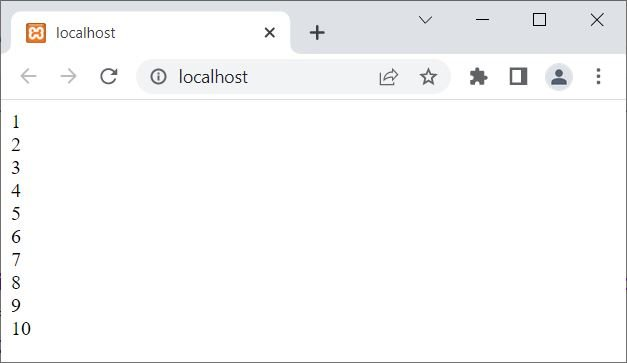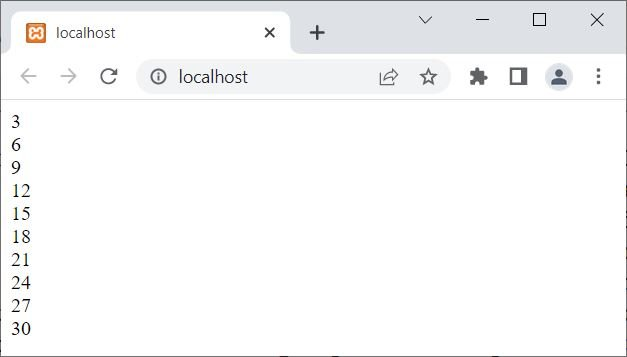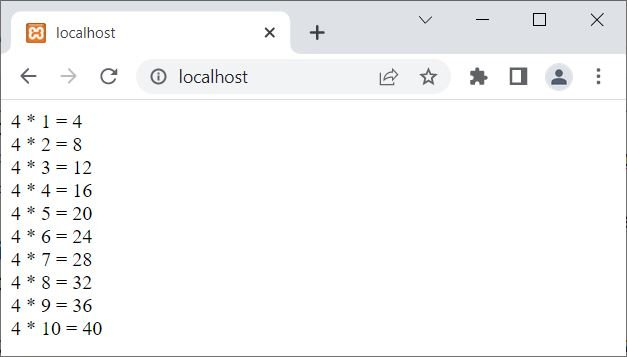# for in PHP with examples

The "for" loop in PHP is used when we need to execute some block of code multiple times.

## PHP for loop syntax

The syntax of the "for" loop in PHP is:

```for(initialize; condition; update)
{
// body of the loop;
}```

The execution of the for loop starts with initialize. This statement executes at the start of the loop and only executes once. This is used to initialize the loop variable, for example: \$x = 1;

Before entering the body of the loop, the condition expression must be evaluated to be true.

If the condition is evaluated to be true, then the "// body of the loop;" will be executed.

After executing the "// body of the loop;", the program flow goes to update part of the loop to update the loop variable.

After updating the loop variable, the condition gets evaluated; as already mentioned, before entering the loop, the condition's expression must be evaluated to true. This process continues until the condition is evaluated as false.

## PHP for loop example

Consider the following PHP code as an example demonstrating the practical use of the "for" loop.

```<?php
for(\$x=1; \$x<=10; \$x++)
echo \$x, "<BR>";
?>```

The output is:The number of times the block of code inside the loop will be executed depends upon how many times the condition of the loop evaluates to be true. In the above example, since the condition \$x<=10; evaluates to be true ten times, the statement echo \$x, "<BR>"; has been executed ten times.

The above program can also be written as:

```<?php
for(\$x=1; \$x<=10; \$x++)
{
echo \$x;
echo "<BR>";
}
?>```

Here is another example of a for loop along with its output and in-depth description:

```<?php
for(\$num=3; \$num<=30; \$num=\$num+3)
{
echo \$num;
echo "<BR>";
}
?>```

The output produced by the above PHP example on the for loop is shown in the snapshot given below:The dry run of the above example is:

• The value 3 has been initialized to the variable \$num, as initially the first statement (initialize) always gets executed first and only once.
• Now that the condition \$num<=30 or 3<=30 evaluates to true.
• Therefore, program flow goes inside the loop.
• And using the two "echo" statements, first the value of \$num gets printed, then a line break will be inserted using the BR tag.
• Now the program flow goes to the update part and will execute the statement available there, which is \$num = \$num + 3.
• The value of \$num will increase by 3 using the update statement. Therefore, now \$num = 6.
• Again, the condition \$num<=30 will be evaluated with the new value of \$num.
• That is, the condition \$num<=30 or 6<=30 evaluates to true again.
• Therefore, program flow again goes inside the loop.
• And the value of \$num, which is 6, will be printed on the output, followed by a new line or line break.
• Again, the value of \$num will be incremented by 3, using the update statement. Now \$num=9.
• The condition \$num<=30 or 9<=30 evaluates to true for the third time.
• Therefore, program flow again goes inside the loop for the third time.
• This process continues until the condition is evaluated as false.

You can also use multiple initialize, update, and/or condition statements. For example, in the following example, I'm going to use two initialization codes for the initialize part of the loop:

```<?php
for(\$num=4, \$i=1; \$i<=10; \$i++)
echo "\$num * \$i = ", \$num*\$i, "<BR>";
?>```

The output of this example is shown in the snapshot given below:Note: When using multiple conditional statements for a condition, the execution of the loop stops when any of the conditional statements evaluates to be false.

### Advantages of the for loop in PHP

• Execution of the loop is completely under your control thanks to the "for" loop. The number of iterations, the iteration step, and additional conditions can all be controlled by developers.
• Efficiency: When the number of iterations is known in advance, the "for" loop is more effective than other loop types. Due to the fact that it only evaluates the loop condition once, it performs better than the "while" and "do-while" loops.
• Consistency: The "for" loop's structure is predictable, which makes it simpler to read and comprehend. Its structure is well-known to most developers, and it is frequently used in programming.

### Disadvantages of the for loop in PHP

• Complexity: Using the "for" loop can be challenging, particularly for newcomers. The initial value, the test condition, and the step to increase or decrease the loop variable must all be specified by developers.
• Lengthy syntax: When additional conditions are added to the "for" loop's syntax to control the loop's execution, it can become quite long. This could make the code harder to read and maintain.
• Risk of infinite loops: If the loop condition is not clearly defined, the "for" loop may result in infinite loops. This could cause the script to use a lot of resources and even bring the server to a halt.
• Limited adaptability: The "for" loop works best when the number of iterations is known ahead of time. It is less suitable for circumstances where the loop's execution depends on unpredictable external variables or conditions.

PHP Online Test

« Previous Tutorial Next Tutorial »